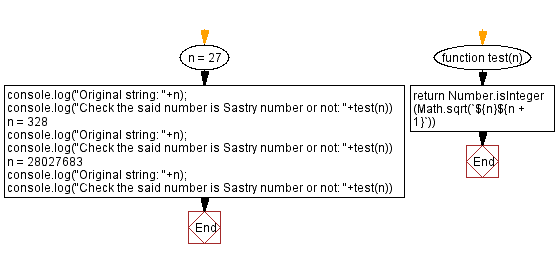# JavaScript: Check a number is Sastry number or not

## JavaScript Math: Exercise-87 with Solution

Write a JavaScript program that checks whether a number (positive integer) is a Sastry number or not.

The number N is a Sastry number when it is concatenated with N+1 and gives a perfect squares. Some Sastry numbers are 183, 328, 528, 715, 6099, 13224, 40495, 106755, 453288, 2066115, 2975208, 22145328, 28027683, 110213248...

Test Data:
(27) -> false
(328) -> true
(28027683) -> true

Sample Solution:

HTML Code:

``````<!DOCTYPE html>
<html>
<meta charset="utf-8">
<title>JavaScript program to Check a number is Sastry number or not</title>
<body>

</body>
</html>
```
```

JavaScript Code:

``````function test(n){
return Number.isInteger(Math.sqrt(`\${n}\${n + 1}`))
}
n = 27
console.log("Original string: "+n);
console.log("Check the said number is Sastry number or not: "+test(n))
n = 328
console.log("Original string: "+n);
console.log("Check the said number is Sastry number or not: "+test(n))
n = 28027683
console.log("Original string: "+n);
console.log("Check the said number is Sastry number or not: "+test(n))
```
```

Sample Output:

```Original string: 27
Check the said number is Sastry number or not: false
Original string: 328
Check the said number is Sastry number or not: true
Original string: 28027683
Check the said number is Sastry number or not: true
```

Flowchart:Live Demo:

See the Pen javascript-math-exercise-87 by w3resource (@w3resource) on CodePen.

Improve this sample solution and post your code through Disqus

What is the difficulty level of this exercise?

Test your Programming skills with w3resource's quiz.

﻿